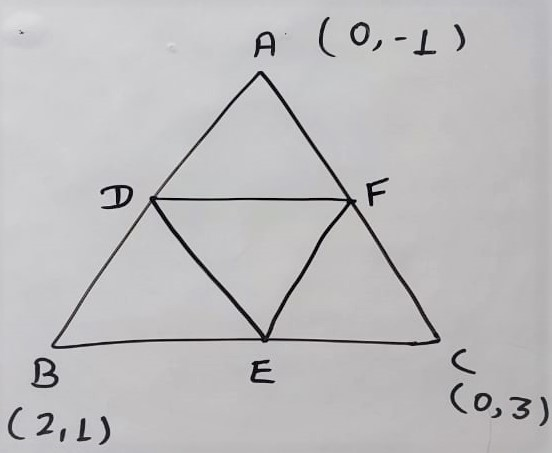## NCERT Solution Exercise 7.3 class 10

Q.1 Find the area of the triangle whose vertices are : (i) (2,3),(-1,0),(2,-4) (ii) (-5,-1),(3,-5),(5,2) Solution: (i) (2,3),(-1,0),(2,-4) Let The co-ordinate of vertices of the triangle are A  (2, 3), B (-1, 0), C(2, -4) x1 = 2 ; y1 = 3 x2 = -1 ; y2 = 0 x3 = 2 ; y3 = …